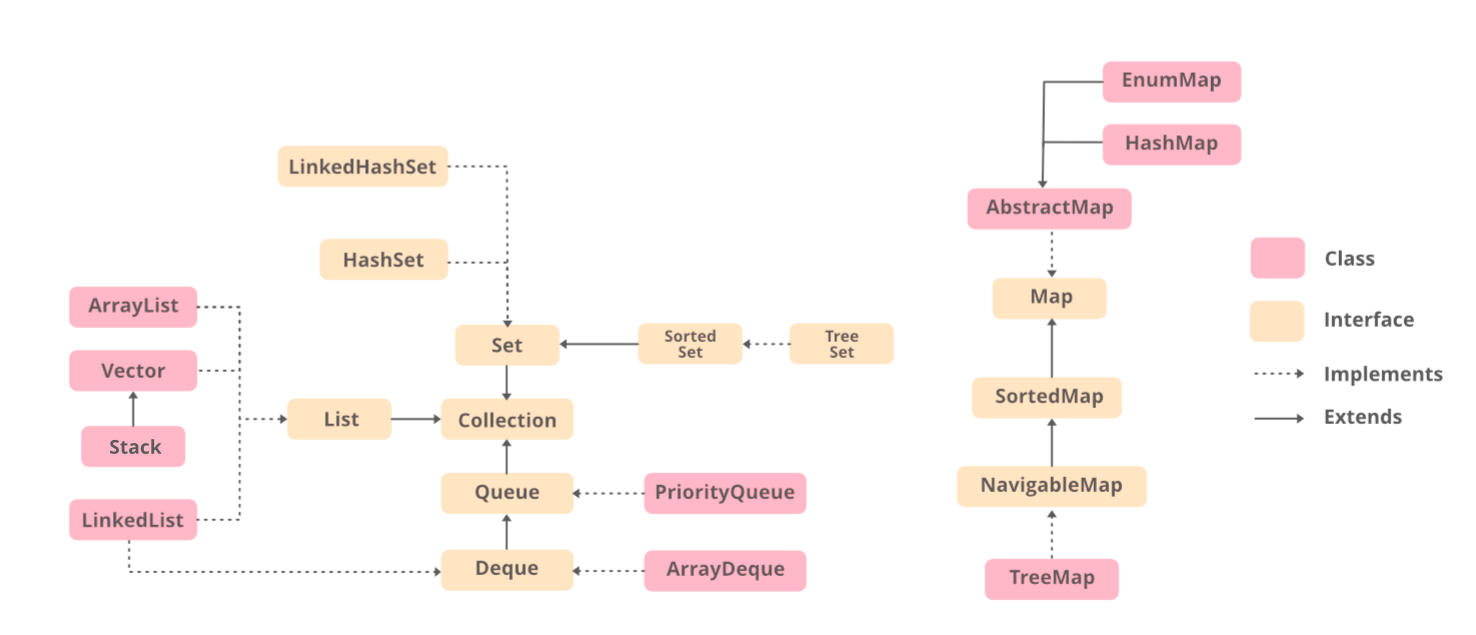Open in App
Not now

# ArrayList vs LinkedList in Java

• Difficulty Level : Easy
• Last Updated : 02 Feb, 2023

An array is a collection of items stored at contiguous memory locations. The idea is to store multiple items of the same type together. However, the limitation of the array is that the size of the array is predefined and fixed. There are multiple ways to solve this problem. In this article, the difference between two classes that are implemented to solve this problem named ArrayList and LinkedList is discussed.ArrayList is a part of the collection framework. It is present in the java.util package and provides us dynamic arrays in Java. Though, it may be slower than standard arrays but can be helpful in programs where lots of manipulation in the array is needed. We can dynamically add and remove items. It automatically resizes itself. The following is an example to demonstrate the implementation of the ArrayList.

Example

## Java

 `// Java program to Illustrate Working of an ArrayList`   `// Importing required classes ` `import` `java.io.*;` `import` `java.util.*;`   `// Main class ` `class` `GFG {`   `    ``// Main driver method ` `    ``public` `static` `void` `main(String[] args)` `    ``{` `        ``// Creating an ArrayList of Integer type` `        ``ArrayList arrli` `            ``= ``new` `ArrayList();`   `        ``// Appending the new elements` `        ``// at the end of the list` `        ``// using add () method via for loops` `        ``for` `(``int` `i = ``1``; i <= ``5``; i++)` `            ``arrli.add(i);`   `        ``// Printing the ArrayList` `        ``System.out.println(arrli);`   `        ``// Removing an element at index 3 ` `        ``// from the ArrayList` `        ``// using remove() method ` `        ``arrli.remove(``3``);`   `        ``// Printing the ArrayList after` `        ``// removing the element` `        ``System.out.println(arrli);` `    ``}` `}`

Output

```[1, 2, 3, 4, 5]
[1, 2, 3, 5]```

LinkedList is a linear data structure where the elements are not stored in contiguous locations and every element is a separate object with a data part and address part. The elements are linked using pointers and addresses. Each element is known as a node. Due to the dynamicity and ease of insertions and deletions, they are preferred over the arrays. The following is an example to demonstrate the implementation of the LinkedList

Note: This class implements the LinkedList Data Structure.

Example

## Java

 `// Java program to Demonstrate Working of a LinkedList`   `// Importing required classes` `import` `java.util.*;`   `// Main class` `class` `GFG {`   `    ``// main driver method` `    ``public` `static` `void` `main(String args[])` `    ``{`   `        ``// Creating an object of the` `        ``// class linked list` `        ``LinkedList object` `            ``= ``new` `LinkedList();`   `        ``// Adding the elements to the object created` `        ``// using add() and addLast() method`   `        ``// Custom input elements` `        ``object.add(``"A"``);` `        ``object.add(``"B"``);` `        ``object.addLast(``"C"``);`   `        ``// Print the current LinkedList` `        ``System.out.println(object);`   `        ``// Removing elements from the List object` `        ``// using remove() and removeFirst() method` `        ``object.remove(``"B"``);` `        ``object.removeFirst();`   `        ``System.out.println(``"Linked list after "` `                           ``+ ``"deletion: "` `+ object);` `    ``}` `}`

Output:

```[A, B, C]